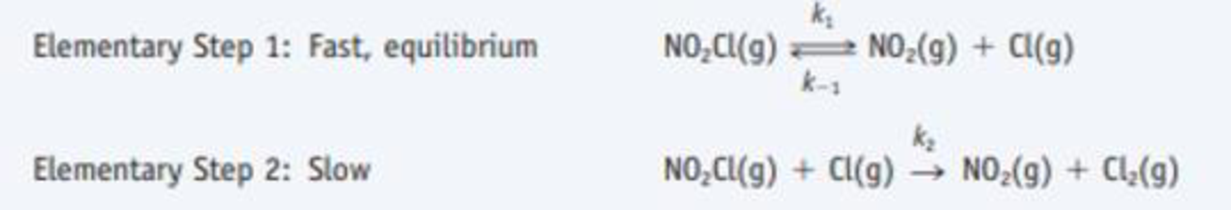Chapter 14.7, Problem 14.14CYU

Chapter
Section
Textbook Problem

One possible mechanism for the decomposition of nitryl chloride, NO2CI, isWhat is the overall reaction? What rate law would be derived from this mechanism? What effect does increasing the concentration of the product NO2 have on the reaction rate?

Interpretation Introduction

Interpretation: The rate law, overall reaction, effect of increasing concentration of the product on reaction rate has to be given.

Concept Introduction:

The rate of reaction is the quantity of formation of product or the quantity of reactant used per unit time.  The rate of reaction doesn’t depend on the sum of amount of reaction mixture used.

The raise in molar concentration of product of a reaction per unit time or decrease in molarity of reactant per unit time is called rate of reaction and is expressed in units of mol/(L.s) .

The variation in concentration of reaction or product over a certain interval of time is called average reaction rate.

The equation that relates the reaction rate to the reactants concentrations that is raised to various powers is called as rate law.

Rate law can be determined by the slow step or otherwise called as rate-determining step.

Explanation

The overall reaction rate law and the effect is given as,

Overall reaction:

Step1:fast,equilibriumNO2Cl(g)  k-1k1NO2(g) + Cl(g)Step2:slowNO2Cl(g) + Cl(g) k2NO2(g) + Cl2(g)_overallreaction:2NO2Cl(g)     ®2NO2(g)   + Cl2(g)_

The overall rate of the given chemical reaction is k[NO]2[O2]

Overall rate of reaction:

Step1:fast,equilibriumNO2Cl(g)  k-1k1NO2(g) + Cl(g)Step2:slow

Still sussing out bartleby?

Check out a sample textbook solution.

See a sample solution

The Solution to Your Study Problems

Bartleby provides explanations to thousands of textbook problems written by our experts, many with advanced degrees!

Get Started